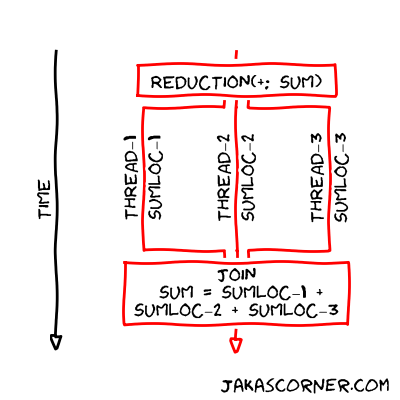When I was researching for this article, I stumbled into this Wikipedia web page. It shows many meanings of a reduction. I clicked on several entries from this page and I realized that a reduction simplifies something complex into something which is understandable and uncomplicated.

Therefore, we can argue that the following example is a reduction

It simplifies the long expression

into something shorter – `sum`. A similar argument argues that also the following example is a reduction

It reduces the long expression into the simpler one.

## Loops

In C++, we can write the previous examples with for loops:

and

In fact, if we have an appropriate operation `o`, we can rewrite

into a for loop

## OpenMP and reduction

OpenMP is specialized into parallelization of for loops. But a parallelization of the previous for loops is tricky. There is a shared variable (`sum` / `product` / `reduction`) which is modified in every iteration. If we are not careful when parallelizing such for loops, we might introduce data races.

For example, if we would parallelize the previous for loops by simply adding

we would introduce a data race, because multiple threads could try to update the shared variable at the same time.

But for loops which represent a reduction are quite common. Therefore, OpenMP has the special `reduction` clause which can express the reduction of a for loop.

In order to specify the reduction in OpenMP, we must provide

• an operation (`+` / `*` / `o`)
• and a reduction variable (`sum` / `product` / `reduction`). This variable holds the result of the computation.

For example, the following for loop

can be parallelized with the `reduction` clause where the operation is equal to `+` and the reduction variable is equal to `sum`:

## Operators and variables

OpenMP is able to perform a reduction for the following operators

Let `o` be one of the previous operators and let `x` be a reduction variable. Further, let `expr` be an expression which does not depend on `x`. OpenMP specifies which statements are supported in the definition of the reduction. This statements occur in the body of the for loop. The statements are

• `x = x o expr`.

For example, if `o == +` and `x == sum` then the statement is `sum = sum + expr`.

• `x o= expr`.

For example, if `o == +` and `x == sum` then the statement is `sum += expr`.

• `x = expr o x`.

In this case the operator `o` is not allowed to be `-`.

There are some additional supported statements if the operator is `+` or `-`. They are

• `x++`,
• `++x`,
• `x--`,
• `--x`.

If the reduction operator `o` is one of the operators listed above and if the statement for the reduction variable `x` is one of the statements listed above, then we can express the reduction with the loop construct:

More information about the specification of the reduction clause is available in the OpenMP specification on the page 201.

## Implementation

How does OpenMP parallelize a for loop declared with a reduction clause? OpenMP creates a team of threads and then shares the iterations of the for loop between the threads. Each thread has its own local copy of the reduction variable. The thread modifies only the local copy of this variable. Therefore, there is no data race. When the threads join together, all the local copies of the reduction variable are combined to the global shared variable.

For example, let us parallelize the following for loop

and let there be three threads in the team of threads. Each thread has `sumloc`, which is a local copy of the reduction variable. The threads then perform the following computationsWe learned how to use the `reduction` clause. We looked at the specifications of the clause and familiarized ourselves with its implementational details.